# Dependency Engine for Deep Learning

We always want deep learning libraries to run faster and scale to larger datasets. One natural approach is to see if we can benefit from throwing more hardware at the problem, as by using multiple GPUs simultaneously.

Library designers then ask: How can we parallelize computation across devices? And, more importantly, how can we synchronize computation when we introduce multi-threading? A runtime dependency engine is a generic solution to these problems.

In this document, we examine approaches for using runtime dependency scheduling to accelerate deep learning. We aim to explain how runtime dependency scheduling can both speed up and simplify multi-device deep learning. We also explore potential designs for a generic dependency engine that could be both library- and operation-independent.

Most of the discussion of on this page draws inspiration from the MXNet dependency engine. The dependency tracking algorithm we discuss was primarily developed by Yutian Li and Mingjie Wang.

## Dependency Scheduling

Although most users want to take advantage of parallel computation, most of us are more familiar with serial programs. So one natural question is: how can we write serial programs and build a library to automatically parallelize our programs in an asynchronous way?

For example, in the following code, we can run `B = A + 1` and `C = A + 2` in any order, or in parallel:

``````    A = 2
B = A + 1
C = A + 2
D = B * C
``````

However, it's quite hard to code the sequence manually because the last operation, `D = B * C`, needs to wait for both of the preceding operations to complete before it starts. The following dependency graph/data flow graph illustrates this.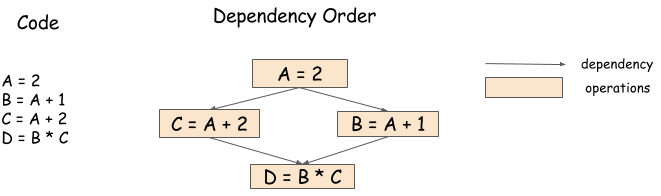A dependency engine is a library that takes a sequence of operations and schedules them according to the dependency pattern, potentially in parallel. So in this example, a dependency library could run `B = A + 1` and `C = A + 2` in parallel, and run `D = B * C` after those operations complete.

## Problems in Dependency Scheduling

A dependency engine relieves the burden of writing concurrent programs. However, as operations become parallelized, new dependency tracking problems arise. In this section, we discuss those problems.

### Data Flow Dependency

Data flow dependency describes how the outcome of one computation can be used in other computations. Every dependency engine has to solve the data flow dependency problem.Because we discussed this issue in the preceding section, we include the same figure here. Libraries that have data flow tracking engines include Minerva and Purine2.

### Memory Recycling

When should we recycle the memory that we allocated to the arrays? In serial processing, this is easy to determine. We simply recycle the memory after the variable goes out of scope. However, as the following figure shows, this is a bit harder in parallel processing.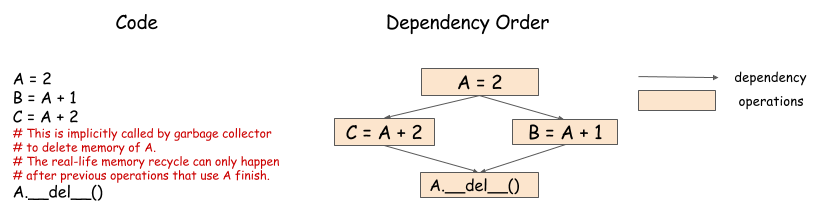In this example, because both computations need to use values from A, we can't recycle the memory until both complete. The engine must schedule the memory recycling operations according to the dependencies, and ensure that they are executed after both `B = A + 1` and `C = A + 2` complete.

### Random Number Generation

Random number generators, which are commonly used in machine learning, pose interesting challenges for dependency engines. Consider the following example: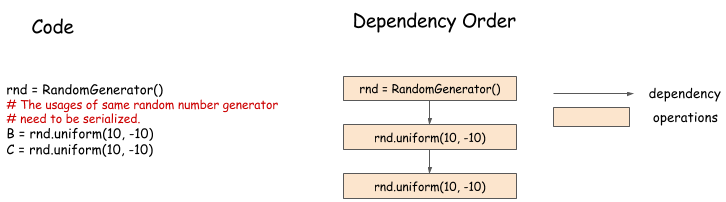In this example, we are generating random numbers in a sequence. Although it seems that the two random number generations can be parallelized, this is usually not the case. A pseudo-random number generator (PRNG) is not thread-safe because it might cause some internal state to mutate when generating a new number. Even if the PRNG is thread-safe, it is preferable to serialize number generation, so we can get reproducible random numbers.

## Case Study: A Dependency Engine for a Multi-GPU Neural Network

In the last section, we discussed the problems we might face in designing a dependency engine. Before thinking about how to design a generic engine to solve those problems, let's consider how a dependency engine can help in multi-GPU training of a neural network. The following pseudocode Python program illustrates training one batch on a two-layer neural network.

``````    # Example of one iteration Two GPU neural Net
data = next_batch()
data[gpu0].copyfrom(data[0:50])
data[gpu1].copyfrom(data[50:100])
# forward, backprop on GPU 0
fc1[gpu0] = FullcForward(data[gpu0], fc1_weight[gpu0])
fc2[gpu0] = FullcForward(fc1[gpu0], fc2_weight[gpu0])
# forward, backprop on GPU 1
fc1[gpu1] = FullcForward(data[gpu1], fc1_weight[gpu1])
fc2[gpu1] = FullcForward(fc1[gpu1], fc2_weight[gpu1])
fc1_weight[cpu].copyto(fc1_weight[gpu0] , fc1_weight[gpu1])
fc2_weight[cpu].copyto(fc2_weight[gpu0] , fc2_weight[gpu1])
``````

In this program, the data 0 to 50 is copied to GPU 0, and the data 50 to 100 is copied to GPU 1. The calculated gradients are aggregated in the CPU, which then performs a simple SGD update, and copies the updated weight back to each GPU. This is a common way to write a parallel program in a serial manner. The following dependency graph shows how it can be parallelized: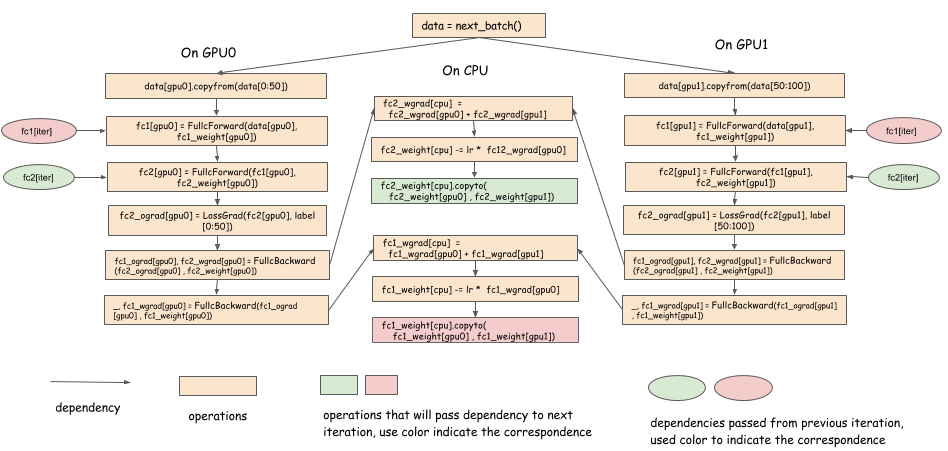Notes:

• The gradient can be copied to the CPU as soon as we get the gradient of a layer.
• The weight can be copied back soon as the weight is updated.
• In the forward pass, we have a dependency on `fc1_weight[cpu].copyto(fc1_weight[gpu0] , fc1_weight[gpu1])` from the previous iteration.
• There is a delay in computation between the last backward pass to layer k and the next forward call to layer k. We can synchronize the weight of layer k in parallel with other computation during this delay.

This approach to optimization is used by multi-GPU deep learning libraries, such as CXXNet. The point is to overlap weight synchronization (communication) with computation. However, it's not easy to do that, because the copy operation needs to be triggered as soon as the backward pass of the layer completes, which then triggers the reduction, updates, etc.

A dependency engine can schedule these operations and perform multi-threading and dependency tracking.

## Designing a Generic Dependency Engine

We hope that you're convinced that a dependency engine is useful for scaling deep learning programs to multiple devices. Now let's discuss how we can design and implement a generic interface for a dependency engine. This solution isn't the only possible design for a dependency engine. It's an example that we think is useful in most cases.

Our goal is to create a dependency engine that is generic and lightweight. Ideally, we'd like the engine that easily plugs into existing deep learning code, and that can scale up to multiple machines with minor modifications. To do that, we need to focus only on dependency tracking, not on assumptions about what users can or can't do.

Here's a summary of goals for the engine:

• The engine should not be aware of what operations it performs, so that users can perform any operations they define.
• It should not be restricted in what type of objects it can schedule.
• We should be able to schedule dependencies on GPU and CPU memory.
• We should be able to track dependencies on the random number generator, etc.
• The engine should not allocate resources. It should only track dependencies. Users can allocate their own memory, PRNG, etc.

The following Python snippet provides an engine interface that might help us reach our goal. Note that a real implementation will be closer to the metal, typically in C++.

``````    class DepEngine(object):
def new_variable():
"""Return a new variable tag
Returns
-------
vtag : Variable Tag
The token of the engine to represent dependencies.
"""
pass

"""Push the operation to the engine.

Parameters
----------
exec_func : callable
The real operation to be performed.

read_vars : list of Variable Tags
The list of variables this operation will read from.

mutate_vars : list of Variable Tags
The list of variables this operation will mutate.
"""
pass
``````

Because we can't make assumptions about what objects we are scheduling, we ask the user to allocate a virtual tag that is associated with each object to represent what we need to schedule. So, at the beginning, the user can allocate the variable tag, and attach it to each of the objects that we want to schedule.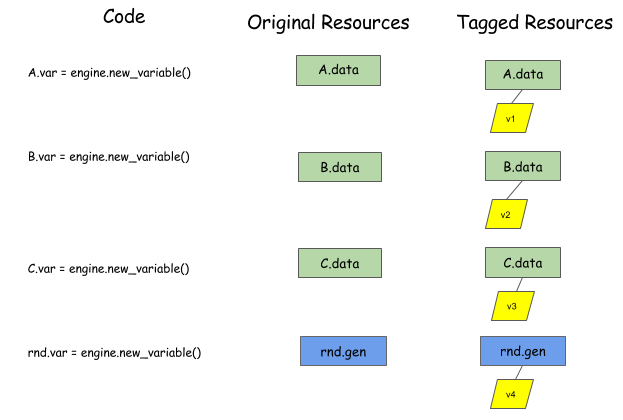The user then calls `push` to tell the engine about the function to execute. The user also needs to specify the dependencies of the operation, using `read_vars` and `write_vars`:

• `read_vars` are variable tags for objects that the operation will read from, without changing their internal state.
• `mutate_vars` are variable tags for objects whose internal states the operation will mutate.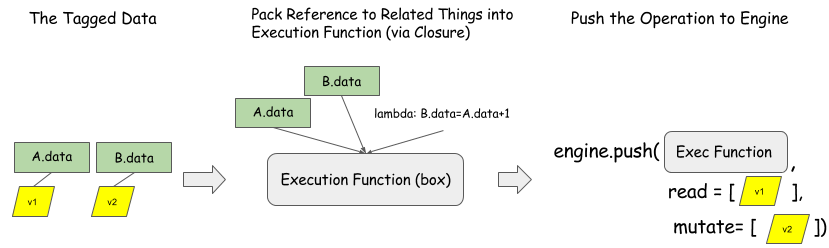The preceding figure shows how to push operation `B = A + 1` to the dependency engine. `B.data` and `A.data` are the allocated space. Note that the engine is only aware of variable tags. Any execution function can be processed. This interface is generic for the operations and resources we want to schedule.

For fun, let's look at how the engine internals work with the tags by considering the following code snippet:

``````    B = A + 1
C = A + 2
A = C * 2
D = A + 3
``````

The first line reads variable `A` and mutates variable `B`. The second line reads variable `A` and mutates variable `C`. And so on.

The engine maintains a queue for each variable, as the following animation shows for each of the four lines. Green blocks represents a read action, while red blocks represent mutations.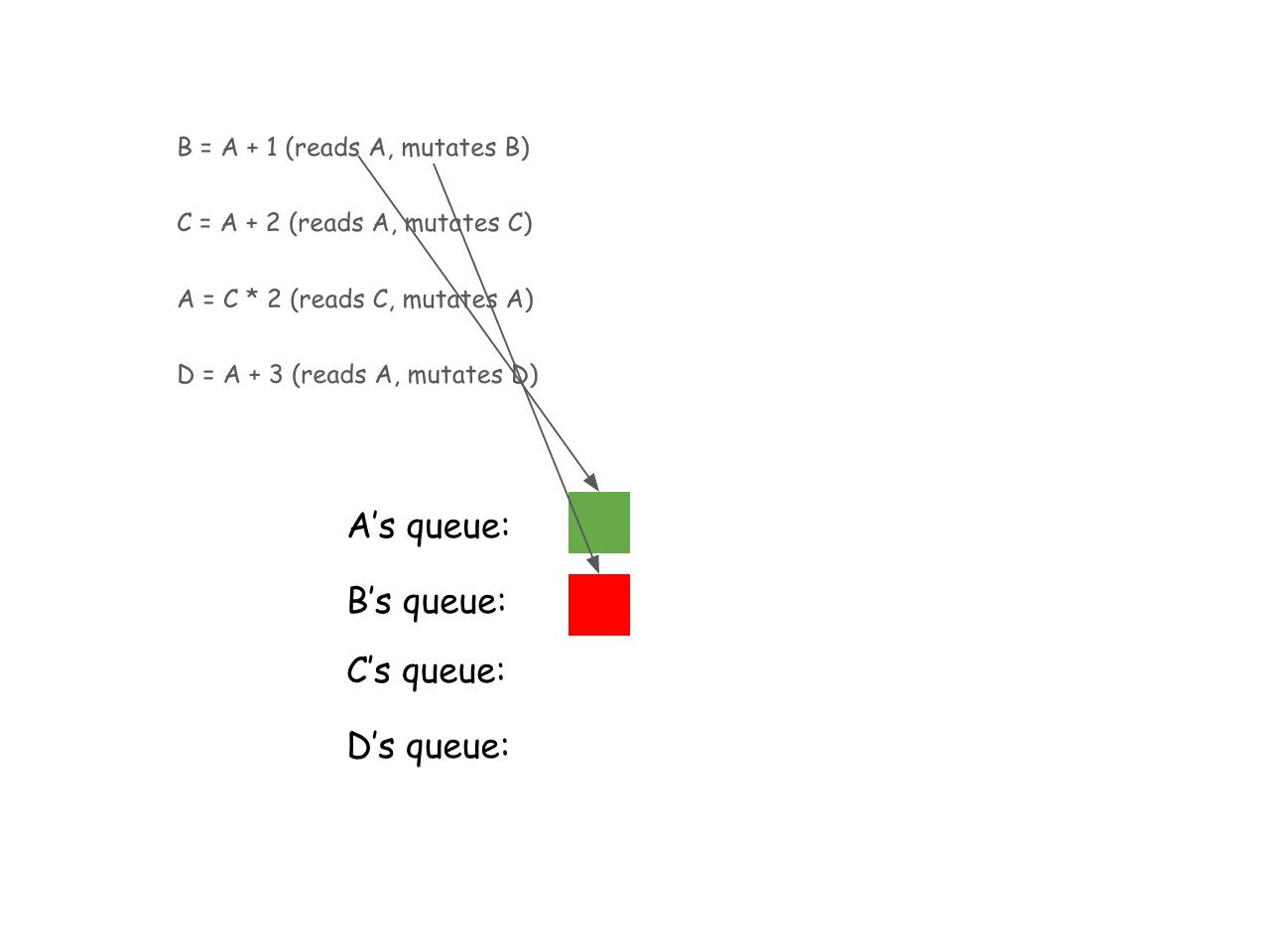Upon building this queue, the engine sees that the first two green blocks at the beginning of `A`'s queue could actually be run in parallel because they are both read actions and won't conflict with each other. The following graph illustrates this point.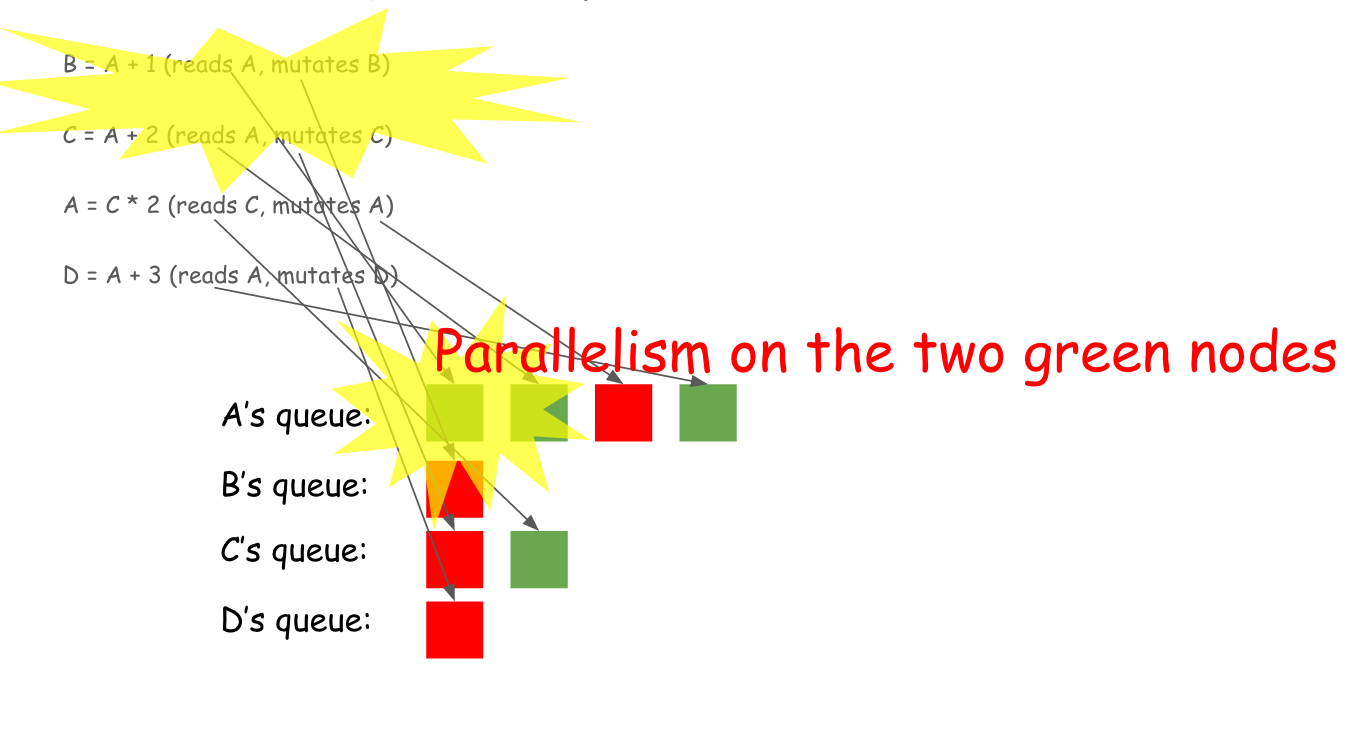One cool thing about all this scheduling is that it's not confined to numerical calculations. Because everything that is scheduled is only a tag, the engine could schedule everything!

The following figure gives a complete push sequence of the programs we mentioned in previous sections.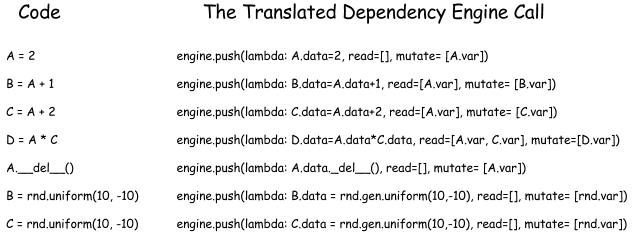### Porting Existing Code to the Dependency Engine

Because the generic interface doesn't control things like memory allocation and which operation to execute, most existing code can be scheduled by the dependency engine in two steps:

1. Allocate the variable tags associated with resources like memory blob, PRNGS.
2. Call `push` with the execution function as the original code to execute, and put the variable tags of corresponding resources correctly in `read_vars` and `mutate_vars`.

## Implementing the Generic Dependency Engine

We have described the generic engine interface and how it can be used to schedule various operations. In this section, we provide a high-level discussion of how to implement such an engine.

The general idea is as follows:

• Use a queue to track all of the pending dependencies on each variable tag.
• Use a counter on each operation to track how many dependencies are yet to be fulfilled.
• When operations are completed, update the state of the queue and dependency counters to schedule new operations.

The following figure illustrates the scheduling algorithm and might give you a better sense of what is going on in the engine.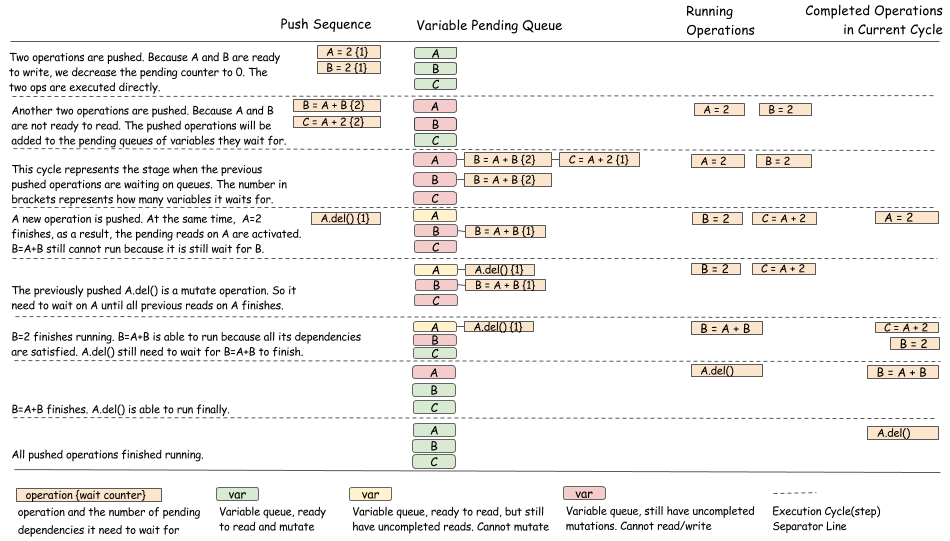Below, we show another example involving random number generators.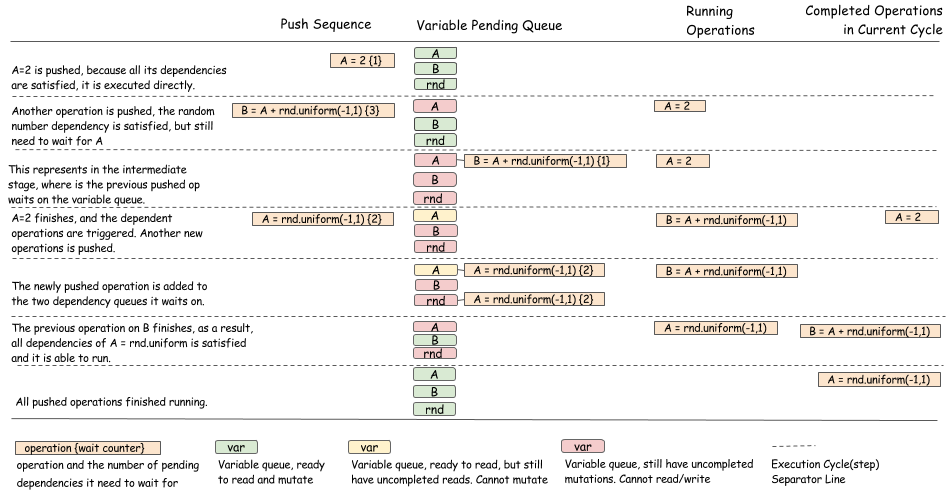As you can see, the purpose of the algorithm is to update pending queues of operations and to make the right state transition when an operation has completed. More care should be taken to make sure the state transitions are done in a way that's safe for threads.

### Separate Dependency Tracking with Running Policy

If you're reading carefully, you might have noticed that the preceding section shows only the algorithm for deciding when an operation can be executed. We didn't show how to actually run an operation. In practice, there can be many different policies. For example, we can either use a global thread-pool to run all operations, or use a specific thread to run operations on each device.

This running policy is usually independent of dependency tracking, and can be separated out as either an independent module or a virtual interface of base-dependency tracking modules. Developing an elegant runtime policy that is fair to all operations and schedules is an interesting systems problem itself.

## Discussion

The design that we discussed in this article isn't the only solution to the dependency tracking problem. It's just one example of how we might approach this. To be sure, some of these design choices are debatable. We'll discuss some of them in this section.

### Dynamic vs. Static

The dependency engine interface discussed in this topic is somewhat dynamic in the sense that the user can push operations one by one, instead of declaring the entire dependency graph (static). Dynamic scheduling can require more overhead than static declarations, in terms of data structure. However, it also enables more flexibility, such as supporting auto parallelism for imperative programs or a mixture of imperative and symbolic programs. You can also add some level of predeclared operations to the interface to enable data structure reuse.

### Mutation vs. Immutable

The generic engine interface presented in this page supports explicit scheduling for mutation. In a typical data flow engine, the data are usually immutable. Working with immutable data has a lot of benefits. For example, immutable data is generally more suitable for parallelization, and facilitates better fault tolerance in a distributed setting (by way of re-computation).

However, immutability presents several challenges:

• It's harder to schedule resource contention problems, as arise when dealing with random numbers and deletion.
• The engine usually needs to manage resources (memory, random number) to avoid conflicts. It's harder to plug in user-allocated space, etc.
• Preallocated static memory isn't available, again because the usual pattern is to write to a preallocated layer space, which is not supported if data is immutable.

Allowing mutation mitigates these issues.

## Source Code of the Generic Dependency Engine

MXNet provides an implementation of the generic dependency engine described in this page. We welcome your contributions.